# HOW TO FACTS AND INFO

### OHM'S LAW

Ohm's Law is Related to Electricity

Ohm's Law is Named After Georg Ohm

### Ohm's Law - A Little Background

Ohm's law: states that the current (I) through a conductor between two points is directly proportional to the voltage (E) across the two points, where I is the current through the conductor in units of amperes, V is the voltage measured across the conductor in units of volts, and R is the resistance of the conductor in units of ohms. Ohm's law also states that the 'R' in this relation is constant, independent of the current.

Resistors: are components that tend to slow down or impede the flow of electricity. The values of resistors are fixed, except for variable resistors. There are numerous types with the most common called carbon resistors. In electrical diagrams or schematics they are represented by a zig-zag picture and the letter 'R' or the Greek symbol "Ω". To measure the resistance of a component you use an ohm meter and measure across the component. This is the resistance or push back to the current flow.

Voltage: is measured across the component you wish to measure. It is the difference in the charge or electrons between two points.

Current: is measured in series with the component. It is the rate at which the charge or voltage is flowing.

Reactive Circuits: include components such as inductors, capacitors or transmissions lines and are generally associated with alternating current or AC circuits. Ohm's law here does not directly apply to this type of circuit. There are no resistors in it. The ac equivalent of resistance is called impedance and is represented by the letter or symbol 'Z.'

In circuit analysis, three equivalent expressions of Ohm's law are used interchangeably:

• V = I * R // Voltage = Current * Resistance
• R = V / I // Resistance = Voltage / Current
• I = V / R // Current = Voltage / Resistance

Each of the above equations can be retrieved from the triangle on the right. Cover the one you need and the two left standing are your answer.

The tool for measuring volts in an electric circuit is called a voltmeter. An ohmmeter is used for measuring resistance. A multimeter can measure several functions including voltage, current, resistance, and temperature.

Resistors In Series: When resistors are connected end-to-end in a circuit (like shown in the picture below) they are said to be in "series." In order to find the total resistance of resistors in series you just add up the value of each resistor. In the example below the total resistance would be R1 + R2.

`Simple Series Resistive Circuit

This is another example of resistors in series. The total value of the resistance across the voltage V is R1 + R2 + R3 + R4 + R5.

`Simple Series Resistive Circuit

Parallel Resistance Circuit:Parallel resistors are resistors that are connected across from each other in an electric circuit. See the picture below. In this picture R1, R2, and R3 are all connected in parallel to each other.

`Simple Parallel Resistive Circuit

When we calculated the series resistance, we totaled the resistance of each resistor to get the value. This makes sense because the current of a voltage across the resistors will travel evenly across each resistor. When the resistors are in parallel this is not the case. Some of the current will travel through R1, some through R2, and some through R3. Each resistor provides an additional path for the current to travel.

In order to calculate the total resistance "R" across the voltage V we use the following formula:

`Parallel Resistance Formula
`Parallel Resistance Example

1/R = ¼ + 1/5 + 1/20
1/R = 5/20 + 4/20 + 1/20
1/R = 10/20 = ½>br> R = 2 Ohms

You can see that the reciprocal of the total resistance is the sum of the reciprocal of each resistance in parallel.

The total resistance is less than the smallest resistor value in parallel circuit. This will always be the case.

Series Parallel Circuits: The idea for solving these types of circuits is to break down smaller parts of the circuit into series and parallel sections. First do any sections that have only series resistors. Then replace those with the equivalent resistance. Next solve the parallel sections. Now replace those with equivalent resistors. Continue through these steps until you reach the solution.

Below is a simple series parallel circuit:

`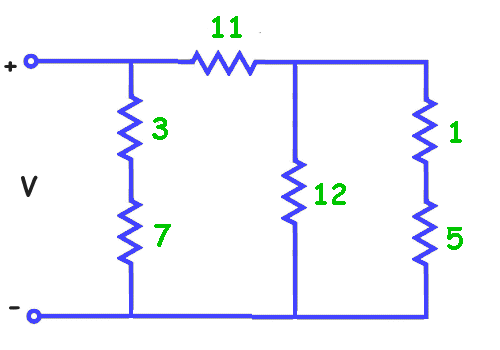Series Parallel Resistance Circuit

First we will total the two series resistors on the right (1 + 5 = 6) and on the left (3 + 7 = 10). Now we have reduced the circuit.

`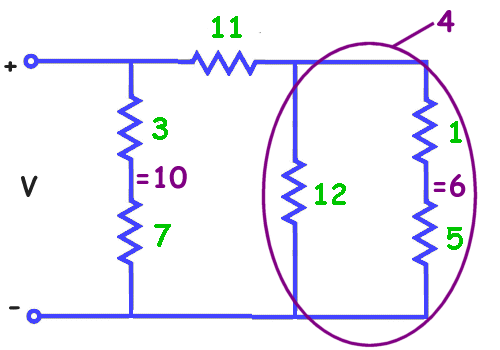Series Parallel Resistance Circuit Problem

We see on the right that the total resistance 6 and the resistor 12 are now in parallel. We can solve for these parallel resistors to get the equivalent resistance of 4.

1/R = 1/6 + 1/12
1/R = 2/12 + 1/12
1/R = 3/12 = ¼
R = 4

The new circuit diagram is shown below:

`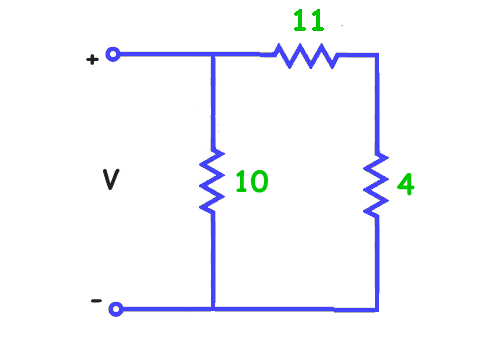Series Parallel Resistance Circuit Problem

From this circuit we solve for the series resistors 4 and 11 to get 4 + 11 = 15. Now we have two parallel resistors, 15 and 10.

The equivalent resistance across V is 6 ohms.

### Simple Electrical Circuit and Ohm's Law Wheel and Triangle

`Simple Electrical Circuit
`Ohm's Law Triangle
`Ohm's Law Wheel

Formula Wheel With PIE: If you look at the wheel above you will see it's divided into four sections. The four are Amps (I) for current, Voltage (E) for volts, Ohms (R) for resistance and a new one, Watts (P) for power.

It looks complicated but using it is really simple.

1. Know what you're trying to solve for, example: voltage or E.
2. What values you already have.
3. Find the section that all your values match.
4. Solve the problem.

Short-Cut Formulas

For resistors in parallel: If all the resistors are of equal value count the resistors and divide that into the value of one resistor. Example: you have ten resistors that are all 100 ohms. Dividing 100 by 10 gives you 10 ohms for Total Resistance.

Another way of saying the above is: If the two resistances or impedances in parallel are equal and of the same value, then the total or equivalent resistance, RT is equal to half the value of one resistor. That is equal to R/2 and for three equal resistors in parallel, R/3, etc. Note that the equivalent resistance is always less than the smallest resistor in the parallel network so the total resistance, RT will always decrease as additional parallel resistors are added.

A Pair Of Resistors - if you have 2 resistors in parallel the the following applies:

1. Multiply the resistances. Example: Two resistors in parallel, 100 and 200 ohms. 100 x 200 = 20,000
2. Add the resistances. Example: 100 + 200 = 300
3. Divide the result in Step 1 by the result in Step 2. This gives you the total resistance. Example: 20,000 / 300 = 66.7 ohms.
4. This only works for two (2) resistors!
`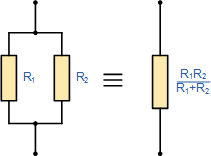Two Equal Resistors in Parallel

### Other LAWS or Rules You Might Find Interesting

Coulomb's Law: The magnitude of the electrostatic force of attraction or repulsion between two point charges is directly proportional to the product of the magnitudes of charges and inversely proportional to the square of the distance between them. The force is along the straight line joining them. If the two charges have the same sign, the electrostatic force between them is repulsive; if they have different signs, the force between them is attractive.

Kirchhoff's Laws (Voltage and Current):
Current Law: The algebraic sum of current into any junction is zero.
The sum of current into a junction equals the sum of current out of the junction.
Since current is the flow of electrons through a conductor, it cannot build up at a junction, meaning that current is conserved: What goes in must come out. Picture a well-known example of a junction: a junction box. These boxes are installed on most houses. They are the boxes that contain the wiring through which all electricity in the home must flow.

If you had a junction of four conductors (wires), the currents v2 and v3 are flowing into the junction, while v1 and v4 flow out of it. In this example, Kirchhoff's Junction Rule yields the following equation:
v2 + v3 = v1 + v4

Voltage Law: Kirchhoff's Voltage Law describes the distribution of electrical voltage within a loop, or closed conducting path, of an electrical circuit. Kirchhoff's Voltage Law states that:

The algebraic sum of the voltage (potential) differences in any loop must equal zero.

The voltage differences include those associated with electromagnetic fields (EMFs) and resistive elements, such as resistors, power sources (batteries, for example) or devices—lamps, televisions, and blenders—plugged into the circuit. Picture this as the voltage rising and falling as you proceed around any of the individual loops in the circuit.

Kirchhoff's Voltage Law comes about because the electrostatic field within an electric circuit is a conservative force field. The voltage represents the electrical energy in the system, so think of it as a specific case of conservation of energy. As you go around a loop, when you arrive at the starting point has the same potential as it did when you began, so any increases and decreases along the loop have to cancel out for a total change of zero. If they didn't, then the potential at the start/end point would have two different values.

When crossing a resistor, the voltage change is determined by the formula:
I*R where I is the value of the current and R is the resistance of the resistor. Crossing in the same direction as the current means the voltage goes down, so its value is negative. When crossing a resistor in the direction opposite the current, the voltage value is positive, so it is increasing.

Voltage and Current Divider Circuits:
The Basics: Voltage Divider and Current Divider are the most common rules applied in practical electronics. As you know, there are two types of combinations in a circuit, they are series and parallel connections. Parallel circuits are also known as current divider circuits because, in these circuits, the current is divided through each resistor. Whereas, series circuits are known as voltage divider circuits because here voltage is divided across all the resistors. Voltage division rule and current division rule are necessary to understand voltage and the current flowing through each resistor. These division rules are used in most common electronic devices.

Voltage Divider:
As we got to know that, a series circuit is known as a voltage divider circuit. It is a circuit which divides the voltage into small parts. So with a power source and two resistors, we can make an easy voltage divider circuit. Here we need to connect two resistors in series combination and then apply a voltage source across the series circuit.

`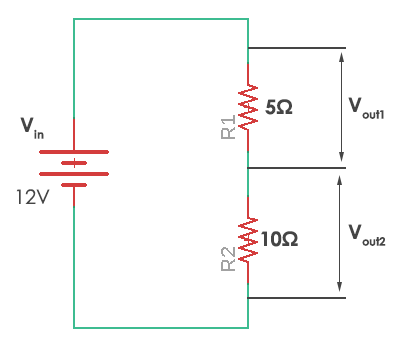Voltage Divider Circuit

In this case, resistor R1 = 5 ohms and resistor R2 = 10 ohms. The voltages Vout1 and Vout2 are divided across the resistors R1 and R2. They can be calculated by a simple voltage dividing equation.

`Voltage Divider Equation

Where Rx is the resistor across which we need to find the voltage and Rtotal is the total resistance (R1+R2) in the circuit. It can be simply calculated by adding all of them as they are connected in series. Thus in the given circuit, the values of the voltage across each resistor are

`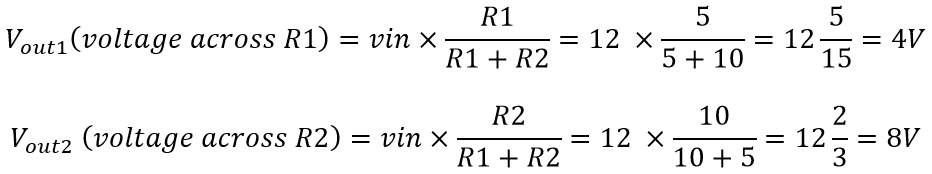Voltage Divider Equation

So you wind up with 4 volts across R1 and 8 volts across R2, hence a simple voltage divider.

### LAWS and Rules - Current Divider

A current divider is a circuit which divides the current into small parts. As we got to know that parallel circuits are current divider circuit. So with a power source and two parallel resistors, we can make an easy current divider circuit. As in the current divider network, here we need to connect two resistors in parallel combination and then apply a current source across the parallel circuit

`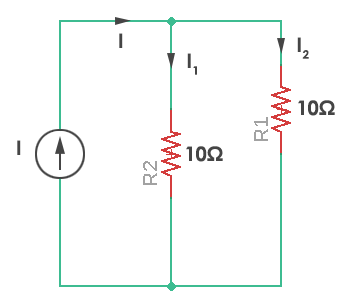Current Divider Circuit

‘I1‘ and ‘I2‘ are the current divided across resistors R1 and R2. They can be calculated by a simple current dividing equation

`Current Divider Equation

I[n]‘ is the required current that flows through the resistor R[n]. R[eq] is the equivalent resistance of the parallel resistors.

Equivalent resistance (Req) is given by:

`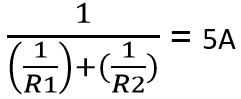Current Divider Equation

So, the current flowing through resistor R1 and R2 would be:

`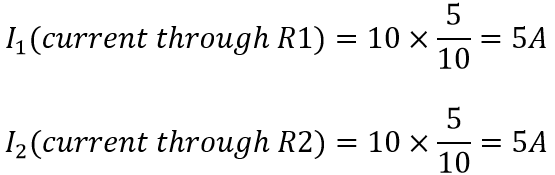Current Divider Equation

Here the resistors are of same value and so current will be divided in exactly half through each resistor. Thus this is known as the current divider circuit. Almost every circuit we come across is either a voltage divider circuit, current divider circuit or it can be both of them.

### LAWS and Rules - Nodal Voltage Analysis

The Nodal Voltage Analysis is a method used to solve the electrical nodes in a network. It is used where it is essential to compute all branch currents.The nodal voltage analysis method determines the voltage and current by using the nodes of the circuit. A node is a terminal or connection of more than two elements.The nodal voltage analysis is commonly used for networks having many parallel circuits with a common terminal ground.This method requires less number of the equation for solving the circuit.

In Nodal Voltage Analysis, Kirchhoff’s Current Law (KCL) is used, which states that the algebraic sum of all incoming currents at a node must be equal to the algebraic sum of all outgoing currents at that node.

It is the method of finding the potential difference between the elements or branches in an electric circuit. This method defines the voltage at each node of the circuit. This method has two type of nodes.They are the non-reference node and the reference node. The non-reference nodes have a fixed voltage, and the reference node is the reference points for all other nodes.

In the nodal method, the number of independent node pair equations needed is one less than the number of junctions in the network. That is if n denotes the number of independent node equations and j is the number of junctions.

n = j – 1

In writing the current expression, the assumptions are made that the node potentials are always higher than the other voltages appearing in the equations.
Let us understand the Nodal Voltage Analysis Method with the help of an example shown below:

`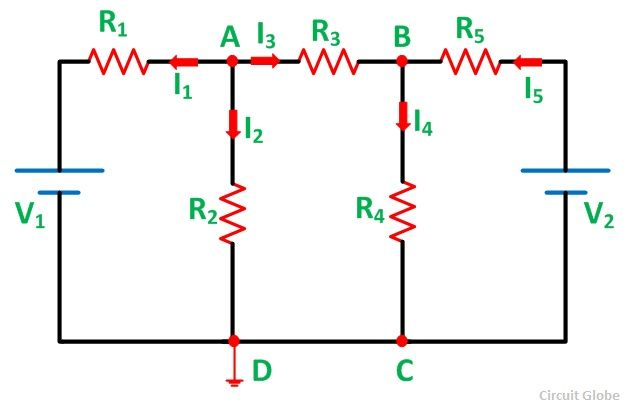Nodal Analysis Schematic

Consider the above circuit. Follow the steps below:

1. Identify various nodes in the given circuit and mark them In the given circuit, we have marked the nodes as A and B.
2. Select one of the nodes as the reference or zero potential nodes at which a maximum number of elements are connected, is taken as reference.
3. In the above figure, node D is taken as the reference node. Let the voltages at nodes A and B be VA and VB respectively.
4. Now apply KCL at the different nodes:
`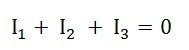Nodal Analysis Equation-KCL

Where

`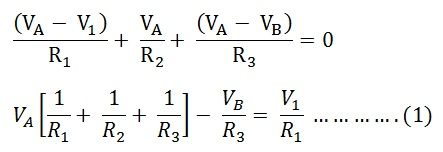Nodal Analysis Equation-KCL

Applying KCL at the node B, we have:

`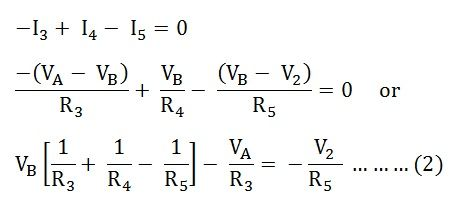Nodal Analysis Equation-KCL

Solving equation (1) and the equation (2) we will get the value of VA and VB. The nodal voltage analysis has the advantage that a minimum number of equations needs to be written to determine the unknown quantities.

### LAWS and Rules = Norton's Theorem

Norton's theorem (aka Mayer–Norton theorem) for DC says:

• Any linear electrical network with voltage and current sources and only resistances can be replaced at terminals A–B by an equivalent current source I[no] in parallel connection with an equivalent resistance R[no].
• This equivalent current I[no] is the current obtained at terminals A-B of the network with terminals A-B short circuited.
• This equivalent resistance R[no] is the resistance obtained at terminals A-B of the network with all its voltage sources short circuited and all its current sources open circuited.

For alternating current (AC) systems the theorem can be applied to reactive impedance as well as resistances.

The Norton equivalent circuit is used to represent any network of linear sources and impedance at a given frequency.

Norton's theorem and Thévenin's theorem, are widely used for circuit analysis simplification and to study circuit's initial-condition and steady-state response.

`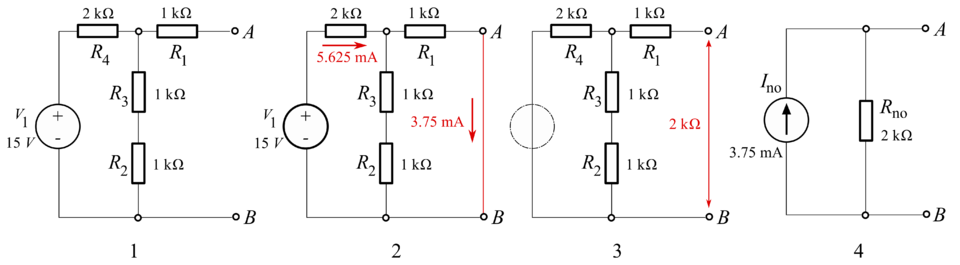Norton Example Circuit
1. The original circuit
2. Calculating the equivalent output current
3. Calculating the equivalent resistance
4. Design the Norton equivalent circuit

In the example, the total current I[total] is given by:

`Norton Formula Equation 1

The current through the load is then, using the current divider rule:

`Norton Formula Equation 2

And the equivalent resistance looking back into the circuit is:

`Norton Formula Equation 3

So the equivalent circuit is a 3.75 mA current source in parallel with a 2 kΩ resistor. [dwg. 4]

### LAWS and Rules - Thévenin's Theorem

As originally stated in terms of DC resistive circuits only, Thévenin's theorem (aka Helmholtz–Thévenin theorem) holds that:

• Any linear electrical network containing only voltage sources, current sources and resistances can be replaced at terminals A-B by an equivalent combination of a voltage source V[th] in a series connection with a resistance R[th].
• The equivalent voltage V[th] is the voltage obtained at terminals A-B of the network with terminals A-B open circuited.
• The equivalent resistance R[th] is the resistance that the circuit between terminals A and B would have if all ideal voltage sources in the circuit were replaced by a short circuit and all ideal current sources were replaced by an open circuit.
• If terminals A and B are connected to one another, the current flowing from A to B will be V[th]/R[th]. This means that R[th] could alternatively be calculated as V[th] divided by the short-circuit current between A and B when they are connected together.

In circuit theory terms, the theorem allows any one-port network to be reduced to a single voltage source and a single impedance.

The theorem also applies to frequency domain AC circuits consisting of reactive and resistive impedance. It means the theorem applies for AC in an exactly same way to DC except that resistances are generalized to impedance.

The equivalent circuit is a voltage source with voltage V[Th] in series with a resistance RTh.

The Thévenin-equivalent voltage V[Th] is the open-circuit voltage at the output terminals of the original circuit. When calculating a Thévenin-equivalent voltage, the voltage divider principle is often useful, by declaring one terminal to be V[out] and the other terminal to be at the ground point.

The Thévenin-equivalent resistance R[Th] is the resistance measured across points A and B "looking back" into the circuit. The resistance is measured after replacing all voltage- and current-sources with their internal resistances. That means an ideal voltage source is replaced with a short circuit, and an ideal current source is replaced with an open circuit. Resistance can then be calculated across the terminals using the formulae for series and parallel circuits. This method is valid only for circuits with independent sources. If there are dependent sources in the circuit, another method must be used such as connecting a test source across A and B and calculating the voltage across or current through the test source.

The replacements of voltage and current sources do what the sources would do if their values were set to zero. A zero valued voltage source would create a potential difference of zero volts between its terminals, regardless of the current that passes through it; its replacement, a short circuit, does the same thing. A zero valued current source passes zero current, regardless of the voltage across it; its replacement, an open circuit, does the same thing.

`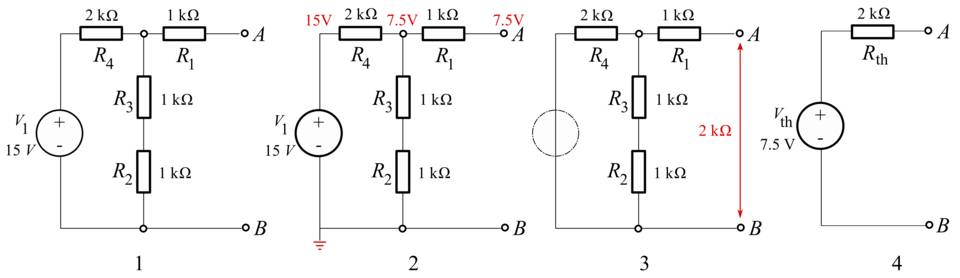Thevenin Circuit Example
1. Original circuit
2. The equivalent voltage
3. The equivalent resistance
4. The equivalent circuit

In the example, calculating the equivalent voltage:

`Thevenin Formula 1

[notice that R1 is not taken into consideration, as above calculations are done in an open-circuit condition between A and B, therefore no current flows through this part, which means there is no current through R1 and therefore no voltage drop along this part]

Calculating equivalent resistance - the total resistance of two parallel resistor

`Thevenin Formula 2

### Resources

1. Wikipedia
2. Electronics For Dummies
3. Fluke
4. Duckster For Kids
5. Sciencing.com
6. Thoughtco.com
7. Codrey.com
8. CircuitGlobe.com
9. Elements OF Radio - By Abraham & William Marcus - 1965
10. Electrical Fundamentals for Technicians - By Robert Shrader - 1969